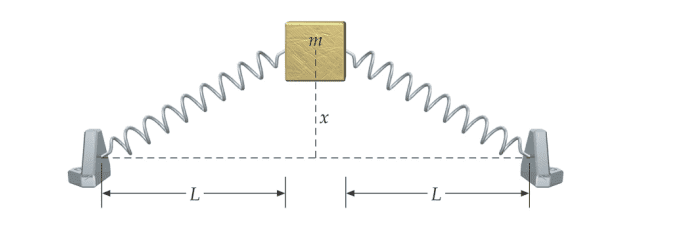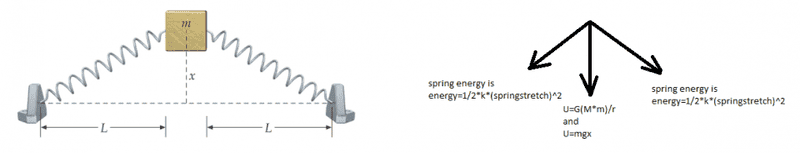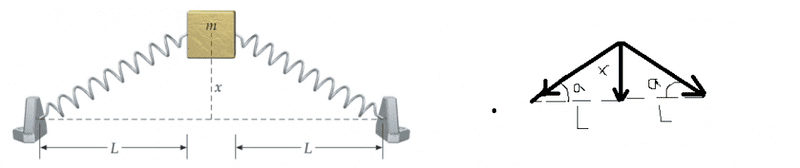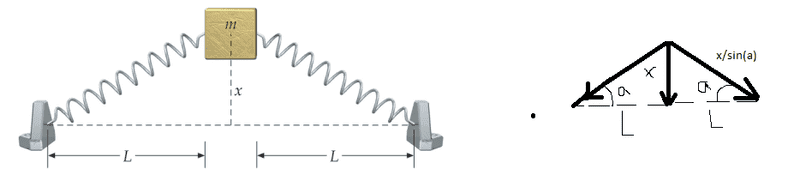# How to calculate the potential energy of a spring?

Hi
I want to calculate the potential energy of the following figure in function of xHere is my attempt :

mgx + 1/2*k*(sqrt(x^2+L^2)-L)=1/2*m*v^2

Is that correct ?

thanks

Last edited by a moderator:

I really suck at physics, but I think that the forces exerted by the two springs should cancel each other out. So, the only thing which comes into play is gravitational potential energy. Someone please correct me if I'm wrong.

Edit: I think have taken only the mass m into account.

berkeman
Mentor
Hi
I want to calculate the potential energy of the following figure in function of x

View attachment 99738

Here is my attempt :

mgx + 1/2*k*(sqrt(x^2+L^2)-L)=1/2*m*v^2

Is that correct ?

thanks
Welcome to the PF.

Not quite right. Please do not deleted the Homework Help Template that you are provided when starting a schoolwork thread here. It helps to organize your thoughts, especially showing the Relevant Equations.

Your "equation" does not make sense in the context of the question you posed. The potential energy does not depend on velocity (why is that on the righthand side of the equation?)

Can you list the Relevant Equations for this problem? If you want to find the PE(x), you will add the gravitational PE to the PE of the stretching springs. Please draw a FBD of the mass in the middle, showing all of the forces on it.
I really suck at physics, but I think that the forces exerted by the two springs should cancel each other out. So, the only thing which comes into play is gravitational potential energy. Someone please correct me if I'm wrong.

Edit: I think have taken only the mass m into account.
No, you are incorrect.

Welcome to the PF.

Not quite right. Please do not deleted the Homework Help Template that you are provided when starting a schoolwork thread here. It helps to organize your thoughts, especially showing the Relevant Equations.

Your "equation" does not make sense in the context of the question you posed. The potential energy does not depend on velocity (why is that on the righthand side of the equation?)

Can you list the Relevant Equations for this problem? If you want to find the PE(x), you will add the gravitational PE to the PE of the stretching springs. Please draw a FBD of the mass in the middle, showing all of the forces on it.

No, you are incorrect.
here is my draw :Is it correct?
thanks

berkeman
Mentor
CloserYou have the gravitational PE correct at mgx.

The PE from the stretched springs has to do with the force in the vertical direction, not in the directions of the springs. Take the vertical component of the spring force(s) to get the PE contribution from the stretched springs.

•CloserYou have the gravitational PE correct at mgx.

The PE from the stretched springs has to do with the force in the vertical direction, not in the directions of the springs. Take the vertical component of the spring force(s) to get the PE contribution from the stretched springs.
mgx = 1/2*k*(x/sin(a) - L)^2 + 1/2*k*(x/sin(a) - L)^2Is it correct?
thanks

berkeman
Mentor
mgx = 1/2*k*(x/sin(a) - L)^2 + 1/2*k*(x/sin(a) - L)^2

View attachment 99745

Is it correct?
thanks
Closer still, but you should be adding mgx to those spring terms. You want an equation for PE(x) = ____________________

I'm also not real clear why you are dividing by the sine of the angle a...

Closer still, but you should be adding mgx to those spring terms. You want an equation for PE(x) = ____________________

I'm also not real clear why you are dividing by the sine of the angle a...
PE(x) = mgx -1/2*k*(x/sin(a) - L)^2 - 1/2*k*(x/sin(a) - L)^2
(x/sin(a) - L) means that the distance of the stretched spring ... So x/sin(a) is total new distance and L is old distance .. So the difference x/sin(a)-L is the distance of the stretched spring ..thanks

berkeman
Mentor
Ah, I get the distance thing now with the x/sin(a)-L. But that just gives you the PE from the force of the spring in the direction of the spring, not in the vertical direction. The horizontal forces of the two springs on the mass cancel out.

Ah, I get the distance thing now with the x/sin(a)-L. But that just gives you the PE from the force of the spring in the direction of the spring, not in the vertical direction. The horizontal forces of the two springs on the mass cancel out.
I do not know how to do ?

haruspex
Homework Helper
Gold Member
The PE from the stretched springs has to do with the force in the vertical direction, not in the directions of the springs. Take the vertical component of the spring force(s) to get the PE contribution from the stretched springs.
No, energy is a scalar. The PE in the springs relates to their extensions. It does not have components.

•No, energy is a scalar. The PE in the springs relates to their extensions. It does not have components.
Do you have any idea how to build the equation of the potential energy for the system
thanks

haruspex
Homework Helper
Gold Member
PE(x) = mgx -1/2*k*(x/sin(a) - L)^2 - 1/2*k*(x/sin(a) - L)^2
Nearly right, but in my experience stretching a spring increases its potential energy.

•Nearly right, but in my experience stretching a spring increases its potential energy.
Can you correct my formula ?
thanks

haruspex
Homework Helper
Gold Member
Can you correct my formula ?
thanks
I can, but you are going to. The equation you wrote would have the springs' contribution to the total PE decreasing when the springs are stretched. What do you think must be wrong?

•I can, but you are going to. The equation you wrote would have the springs' contribution to the total PE decreasing when the springs are stretched. What do you think must be wrong?
is it the signs ?
PE(x) = mgx +1/2*k*(x/sin(a) - L)^2 + 1/2*k*(x/sin(a) - L)^2

haruspex
Homework Helper
Gold Member
is it the signs ?
PE(x) = mgx +1/2*k*(x/sin(a) - L)^2 + 1/2*k*(x/sin(a) - L)^2
Yes.

•Yes.
So now it is correct formula ?

Yes.
thank you very much

SammyS
Staff Emeritus
Homework Helper
Gold Member
here is my draw :

View attachment 99744

Is it correct?
thanks
It strikes me that from that figure, the block may be moving on a horizontal surface.

It strikes me that from that figure, the block may be moving on a horizontal surface.
Is that the force of the block in function of x is : F(x) = k*(x/sin(a) - L) + k*(x/sin(a) - L)
and the kinetic energy in function of x is : KE(x)= 1/2*m*v^2 + 1/2*m*v^2 (I cannot express it in x)
Is that correct ?
thanks

haruspex
Homework Helper
Gold Member
Is that the force of the block in function of x is : F(x) = k*(x/sin(a) - L) + k*(x/sin(a) - L)
and the kinetic energy in function of x is : KE(x)= 1/2*m*v^2 + 1/2*m*v^2 (I cannot express it in x)
Is that correct ?
thanks
Why the two 1\2 mv2 terms?
You probably need to express it in terms of dx/dt.

Why the two 1\2 mv2 terms?
You probably need to express it in terms of dx/dt.
So you mean that this F(x) = k*(x/sin(a) - L) + k*(x/sin(a) - L) is correct !!
and KE(x)= 1/2*m*v^2 + 1/2*m*v^2 = KE(x)= 1/2*m*(dx/dt)^2 + 1/2*m*(dx/dt)^2
Is it like that?
thank you very much

Why didn't you use vectors if you want the net force on the object?

I think he means, Why do you have two 0.5 m v^2? What does the second one represent?

I guess I know what the mentor meant by getting its components.. Because the vertical is doing positive work and the horizontal should have a work of 0 J because it doesn't move horizontally( Springs forces cancel each other if they have the same spring constant).

So If you use the vertical component but you have a variable that changes over time which is theta but both ways should yield in the same result right?

Last edited:
Why didn't you use vectors if you want the net force on the object?

I think he means, Why do you have two 0.5 m v^2? What does the second one represent?
We have two springs so the kinetic energy should be double as much ,, is not that?
thanks

We have two springs so the kinetic energy should be double as much ,, is not that?
thanks
Eh...?
From what I know we use f = kd only if the springs are mass-less... So you they don't actually have kinetic energy.

What you should substitute there is the mass of the object not the springs

Eh...?
From what I know we use f = kd only if the springs are mass-less... So you they don't actually have kinetic energy.

What you should substitute there is the mass of the object not the springs
Can you write the kinetic energy equation here?

haruspex
Homework Helper
Gold Member
We have two springs so the kinetic energy should be double as much ,, is not that?
thanks
The kinetic energy is possessed by the mass m, and there is only one of those. The springs are considered massless. The mass does not care how it gets its KE, whether from one spring or two.

The kinetic energy is possessed by the mass m, and there is only one of those. The springs are considered massless. The mass does not care how it gets its KE, whether from one spring or two.
can you write the equation here ?
thanks

haruspex
Homework Helper
Gold Member
can you write the equation here ?
thanks
To recap:
In post #16 you had the right expression for the potential energy in the springs.

Unless you have some good reason to suppose it is in the vertical plane, go with Sammy's suggestion that is is horizontal, so discard any gravitational term.

In post #21 your force equation is quite wrong. You cannot add forces acting in different directions as though they are scalars. If you want the net force from the springs then you need to consider the x and y components of the forces. (This is the path berkeman was going down, but he wrongly tried to apply that to the PE of the springs instead of to their tensions.). But I don't think you need to worry about forces anyway, just concentrate on energy.

In post #21, your KE expression had two copies of 1/2 mv2. There is one mass m moving at speed v, not two.

From all that, see if you can put together the correct expression for the total energy.

To recap:
In post #16 you had the right expression for the potential energy in the springs.

Unless you have some good reason to suppose it is in the vertical plane, go with Sammy's suggestion that is is horizontal, so discard any gravitational term.

In post #21 your force equation is quite wrong. You cannot add forces acting in different directions as though they are scalars. If you want the net force from the springs then you need to consider the x and y components of the forces. (This is the path berkeman was going down, but he wrongly tried to apply that to the PE of the springs instead of to their tensions.). But I don't think you need to worry about forces anyway, just concentrate on energy.

In post #21, your KE expression had two copies of 1/2 mv2. There is one mass m moving at speed v, not two.

From all that, see if you can put together the correct expression for the total energy.
the kinetic energy in function of x is : KE(x)= 1/2*m*v^2= 1/2*m*(dx/dt)^2
Is it correct now?

haruspex
Homework Helper
Gold Member
the kinetic energy in function of x is : KE(x)= 1/2*m*v^2= 1/2*m*(dx/dt)^2
Is it correct now?
Yes. So what is the total energy?

Yes. So what is the total energy?

total energi = 1/2*m*(dx/dt)^2 + mgx +1/2*k*(x/sin(a) - L)^2 + 1/2*k*(x/sin(a) - L)^2
the kinetic energy is 1/2*m*(dx/dt)^2 ... Can we rewrite the kinetic energy equation in function of x instead of dx ?

haruspex
Homework Helper
Gold Member
total energi = 1/2*m*(dx/dt)^2 + mgx +1/2*k*(x/sin(a) - L)^2 + 1/2*k*(x/sin(a) - L)^2
the kinetic energy is 1/2*m*(dx/dt)^2 ... Can we rewrite the kinetic energy equation in function of x instead of dx ?
No, you cannot express it purely in terms of x except by solving the differential equation.
What is the whole question? You originally said you wanted to find the potential energy, which you have done. Why is the KE of interest? Do you need to find the equation of motion?

Also, I see you have reintroduced mgx. That is applicable if the motion is in the vertical plane, but not if it is in the horizontal plane. Do you know which plane it is in? If the question does not state, I would assume it is horizontal, and throw away the mgx term.

No, you cannot express it purely in terms of x except by solving the differential equation.
What is the whole question? You originally said you wanted to find the potential energy, which you have done. Why is the KE of interest? Do you need to find the equation of motion?

Also, I see you have reintroduced mgx. That is applicable if the motion is in the vertical plane, but not if it is in the horizontal plane. Do you know which plane it is in? If the question does not state, I would assume it is horizontal, and throw away the mgx term.
Why horizontal though?

The forces in the horizontal direction cancel each other ( If we assume that both of the springs have a constant of k) and the net force in the y direction. So It will only move vertically...?